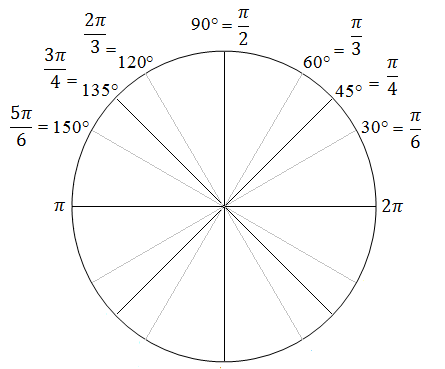Degree/Radian Calculator Enter the angle measure you wish to convert. Type "pi" and the * and / symbols for expressions involving π. Example, enter 3π/2 as either 3*pi/2 or 1.5*pi.      angle = degreesradians

In geometry, there are two ways to measure the size of an angle. In the degrees system, one full revolution is divided into 360 smaller units called degrees. For example, 90° represents 1/4 of a full turn, 60° represents 1/6 of a full turn, etc.

In the radians system, one full revolution is equal to 2π radians, which happens to be the circumference of a unit circle (a circle whose radius is one). For example, half a revolution is π radians, which is equivalent to 180°. A quarter revolution is 2π/4 radians, or 90°.

Although radians may be cumbersome to work with in practical applications, such as construction, they are the preferred unit of angle measurement in calculus and physics. Trigonometric functions whose arguments are in radians have nicer mathematical properties than trig functions whose arguments are in degrees.

You can use the conversion formulas below or the calculator to change degrees into radians and vice versa.

Since there are 2π in 360° the formula for converting radians to degrees is

radians times 360/(2π) = degrees, or

The formula for converting degrees to radians is